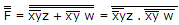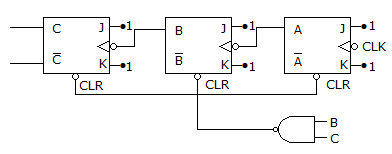# Electronics and Communication Engineering - Digital Electronics

31.

Minimum number of 2-input NAND gates required to implement the function F = (x + y) (Z + W) is

 A. 3 B. 4 C. 5 D. 6

Explanation:

F = (x + y) (z + w) = xy.(z + w)

= xyz + xyw

=minimum no. of 2 input NAND gate.

32.

Which device has one input and many outputs?

 A. Multiplexer B. Demultiplexer C. Counter D. Flip flop

Explanation:

Demultiplexer takes data from one line and directs it to any of its N output depending on the status of its select lines.

33.

 A. it costs less B. it is faster C. it is more accurate D. is uses fewer gates

Explanation:

In look ahead carry adder the carry is directly derived from the gates when original inputs are being added. Hence the addition is fast. This process requires more gates and is costly.

34.

The counter in the given figure isA. Mod 3 B. Mod 6 C. Mod 8 D. Mod 7

Explanation:

When counter is 110 the counter resets. Hence mod 6.

35.

 A. index register value B. sum of the index register value and the operand C. operand D. difference of the index register value and the operand